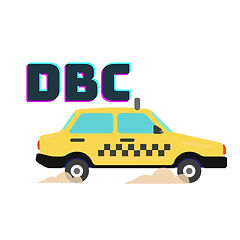# Understanding CAN DBC

## CAN DBC refers to database CAN. In simple terms, the type of data that communicates over a CAN bus can be read and understood using DBC files. DBC is an ASCII based translation, a standard file evolved in the 1990s. Now being used by the automotive industries worldwide. These files can help identify the data within the CAN frame by describing it. As these CAN database, text files contain information for decoding raw CAN bus to physical values. Thus, functioning as a signal library.## For a DBC file, the signal is not an electrical input or output, but it is a physical parameter, such as temperature, engine speed, voltages etc. DBC is the most common way to handle the identification and translation of 8-byte hexadecimal CAN message and raw CAN data. The data in a CAN frame can be broken up into eight one-byte values, sixty-four one-bit values, one sixty-four bit value, or any combination of these. The data field can contain up to 8 bytes of data. A single CAN frame can contain 0 to 64 individual signals (for 64 channels, they would all be binary).

The DBC file contains the following information.

• The CAN ID of the message in which this signal is present

• The position where the signal is present in the CAN message

• The byte order of the signal

• The conversion details of the signal

• Unit of the signalNow let us try to understand this in detail, with the help of an example using Influx Dialog Software

Engine RPM Signal in Message named ECU defined in a DBC.

Message Definition:The message definition contains the following information:

• CAN ID: CAN ID with which the message is transmitted.

• Name of the message.

• Type of CAN Message (CAN Standard/Extended or CAN FD Standard/Extended).

• Data Length Code (DLC) – Length of the Data in the CAN message.

• Transmitter: CAN Node / Control unit from which the message is transmitted.

Signal Definition:The signal definition contains the following information:

Signal Name: Name of the signal present in the message.

Unit: Unit of the physical data present in the signal.

Start Bit: Start Bit of the signal.

Bit Count: Bit count of the signal.

Byte Order: Byte order of the signal, Motorola (Big Endian) / Intel (Little Endian).

Type: Data Type.

Factor: Conversion factor for the signal.

Offset Conversion offset for the signal.

Minimum & Maximum value of the signal.

Defining the position where the signal is present.• The bit position is defined by the start bit, Bit count and the Byte Oder.

• For this signal, the Start bit is 48, the Bit Count is 16, and Motorola's byte order is.

• So from the received raw can message, Out of 64 bits of CAN Data, 16 bit of data starting from 48 to 63 in Motorola byte order is Engine RPM data.Applying conversion formula in the received raw data using information from DBC.

For Example

From the DBC, we have the information that the conversion factor is 0.25, and the offset is 0.

So when we apply it in the conversion formula ((A*raw) +B)

Factor A=0.25, Offset B=0,

0xFFFF is Hexadecimal is 65535 in Decimal

(0.25*65535+0) =16383.75

We know that the unit is RPM from the DBC, so the Physical value is 16383.75 RPM

So, a DBC file helps in understanding what data is communicating through the CAN bus. Doing it manually might look like an impossible task, but software makes it quick and easy. Many Windows applications available for the PC to read a DBC file, including Influx Dialog Plus, Influx Module Analyser, Influx ReXdesk MATLAB Vehicle Network Toolbox from Mathworks, CAN db++ from Vector, and many more. Also, it's possible to read and edit a DBC file with Windows Notepad, but it's a bit complex. Influx Dialog tool is an efficient tool for creating/editing dbc.Training

Influx technology qualified engineers can arrange training related to CAN BUS and its various protocols. For related training, please contact: support_india@influxtechnology.com